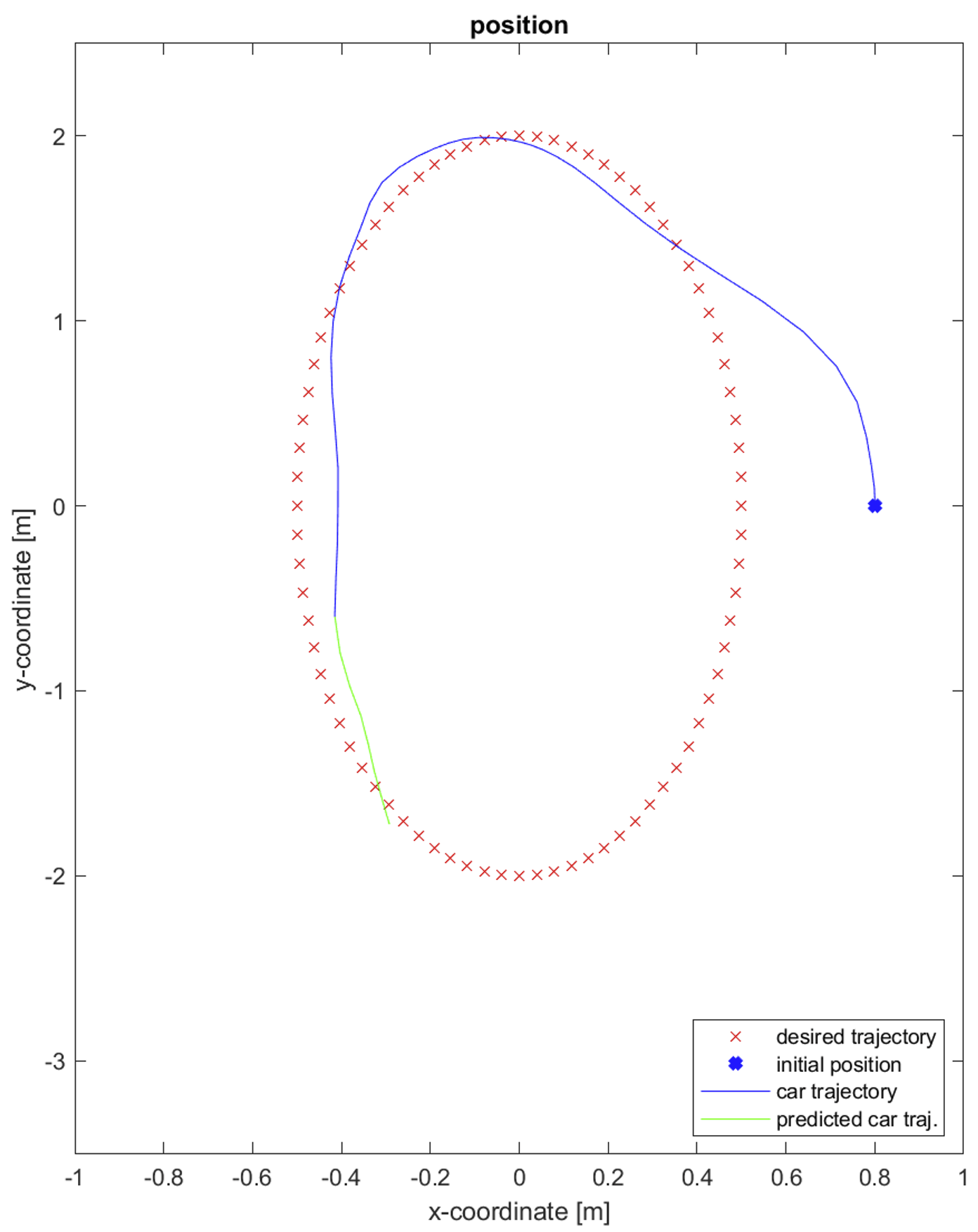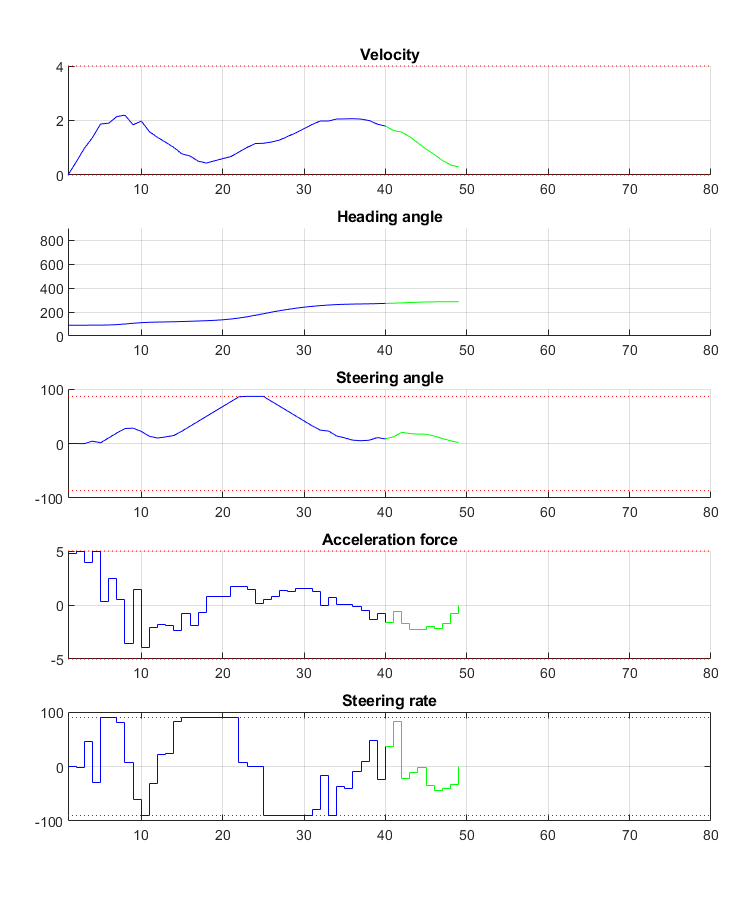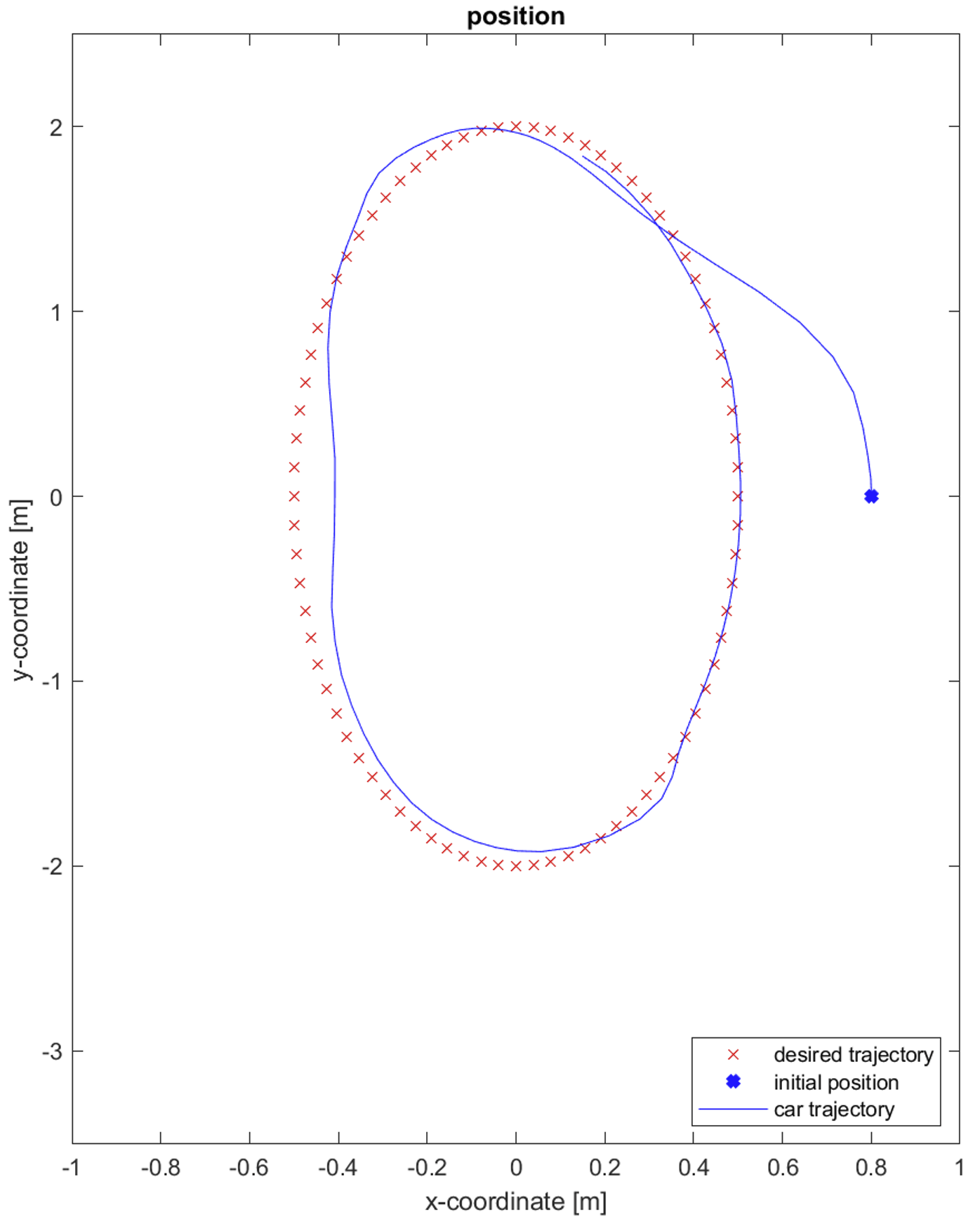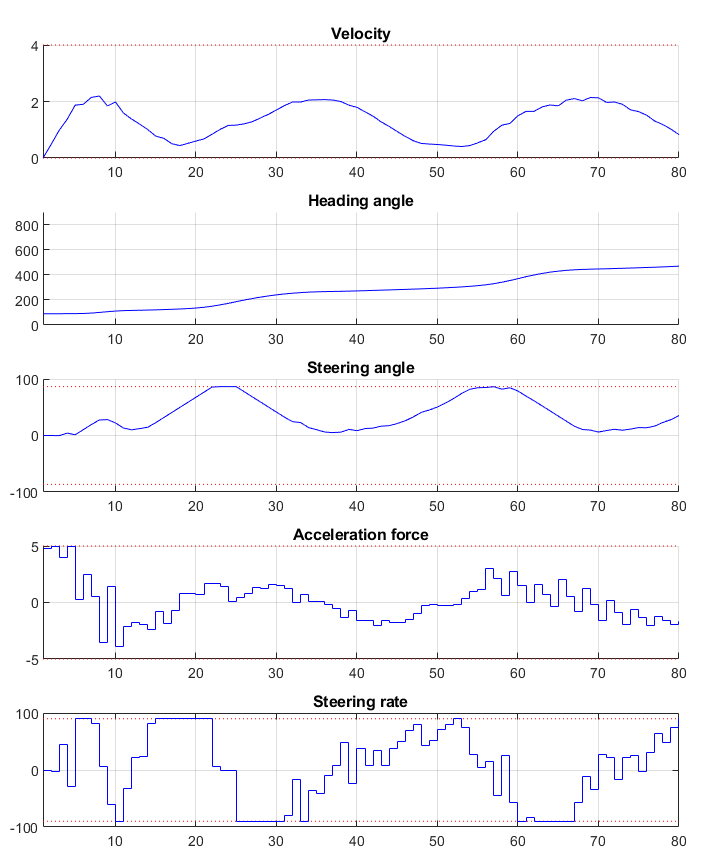# 11.12. High-level interface: Legacy path tracking example (MATLAB & Python)¶

In this example we illustrate the simplicity of the SQP_NLP API on a path-tracking problem. In every simulation step, the predicted trajectory of the car is optimized to follow a set of path points. The example also visualizes how the predicted trajectory changes while the car moves forward.

Note that an improved version of this example is available for MATLAB, see High-level interface: Path tracking example (MATLAB).

We use a kinematic bicycle model described by a set of ordinary differential equations (ODEs):

$\begin{split}\dot{x} & = v \cos(\theta + \beta) \\ \dot{y} & = v \sin(\theta + \beta) \\ \dot{v} & = \frac{F}{m} \\ \dot{\theta} & = \frac{v}{l_r} \sin(\beta) \\ \dot{\delta} &= \phi\end{split}$

with:

$\beta = \arctan\left( \frac{l_r}{l_r + l_f} \tan( \delta)\right)$

The model consists of five differential states: $$x$$ and $$y$$ are the Cartesian coordinates of the car, and $$v$$ is the linear velocity. The angles $$\theta$$ and $$\delta$$ denote the heading angle of the car and its steering angle. Next, there are two control inputs to the model: the acceleration force $$F$$ and the steering rate $$\phi$$. The angle $$\beta$$ describes the direction of movement of the car’s center of gravity relative to the heading angle $$\theta$$. The remaining three constant parameters of the system are the car mass $$m=1\,\mathrm{kg}$$, and the lengths $$l_r=0.5\,\mathrm{m}$$ and $$l_f=0.5\,\mathrm{m}$$ specifying the distance from the car’s center of gravity to the rear wheels and the front wheels, respectively.

The trajectory of the vehicle will be defined as an NLP. First, we define stage variable $$z$$ by stacking the input and differential state variables:

$z = [F,\phi,x,y,v,\theta,\delta]^\top$

You can download the code of this example to try it out for yourself by clicking here (MATLAB) or here (Python).

## 11.12.1. Defining the MPC Problem¶

### 11.12.1.1. Objective¶

In this example the cost function is the same for all stages except for the last stage N. The objective of this example is to follow a set of path points. At runtime, a target position $$p_i$$ for each stage $$i$$ is provided. Each point consists of a x- and a y-coordinate:

$p_i = [p_{i,x}, p_{i,y}]^\top$

The goal is to minimize the distance of the car to these target points. The distance is penalized with quadratic costs. Plus, some small quadratic costs are added to the inputs $$F$$ and $$s$$, i.e.:

$f(z,p_i) = 200 (z_3 - p_{i,x})^2 + 200 (z_4 - p_{i,y})^2 + 0.2 z_1^2 + 0.2 z_2^2$

Since all cost terms are quadratic and summed up, we can formulate the objective as a least squares problem:

$\begin{split}f(z,p_i) & = \frac{1}{2} || r(z,p_i) ||_2^2 \\ r(z,p_i) & = [ \sqrt{200} (z_3 - p_{i,x}), \sqrt{200} (z_4 - p_{i,y}), \sqrt{0.2} z_1, \sqrt{0.2} z_2 ]^\top\end{split}$

The stage cost function is coded in MATLAB and Python as the following function:

model.LSobjective = @LSobj;

function [r] = LSobj(z,currentTarget)
% z = [F,phi,xPos,yPos,v,theta,delta]
% currentTarget = point on path that is to be headed for

r = [sqrt(200.0)*(z(3)-currentTarget(1)); % costs for deviating from the path in x-direction
sqrt(200.0)*(z(4)-currentTarget(2));  % costs for deviating from the path in y-direction
sqrt(0.2)*z(1);                       % penalty on input F
sqrt(0.2)*z(2)];                      % penalty on input phi
end

model = forcespro.nlp.SymbolicModel()  # create empty model
model.objective = obj

def obj(z,current_target):
"""z = [F,phi,xPos,yPos,v,theta,delta]
current_target = point on path that is to be headed for
"""
return (100.0*(z-current_target)**2    # costs on deviating on the path in x-direction
+ 100.0*(z-current_target)**2  # costs on deviating on the path in y-direction
+ 0.1*z**2                        # penalty on input F
+ 0.1*z**2)                       # penalty on input phi


Note that using the model.LSobjective option instead of model.objective allows you to try out the gauss-newton method for the hessian approximation.

For the last stage, the terminal costs are slightly increased by adapting the weighting factors:

$f(z,p_i) = 400 (z_3 - p_{i,x})^2 + 400 (z_4 - p_{i,y)}^2 + 0.4 z_1^2 + 0.4 z_2^2$

The code looks a follows:

model.LSobjectiveN = @LSobjN;

function [r] = LSobjN(z,currentTarget)
% z = [F,phi,xPos,yPos,v,theta,delta]
% currentTarget = point on path that is to be headed for

r = [sqrt(400.0)*(z(3)-currentTarget(1)); % costs for deviating from the path in x-direction
sqrt(400.0)*(z(4)-currentTarget(2));  % costs for deviating from the path in y-direction
sqrt(0.4)*z(1);                       % penalty on input F
sqrt(0.4)*z(2)];                      % penalty on input phi
end

model.objectiveN = objN

def objN(z,current_target):
"""z = [F,phi,xPos,yPos,v,theta,delta]
current_target = point on path that is to be headed for
"""
return (200.0*(z-current_target)**2    # costs on deviating on the path in x-direction
+ 200.0*(z-current_target)**2  # costs on deviating on the path in y-direction
+ 0.2*z**2                        # penalty on input F
+ 0.2*z**2)                       # penalty on input phi


### 11.12.1.2. Matrix equality constraints¶

The matrix equality constraints model.eq in this example result from the vehicle’s dynamics given above. First, the continuous dynamic equations are implemented as follows:

function [xDot] = continuousDynamics(x,u)
% state x = [xPos,yPos,v,theta,delta], input u = [F, phi]

% set physical constants
l_r = 0.5; % distance rear wheels to center of gravity of the car
l_f = 0.5; % distance front wheels to center of gravity of the car
m = 1.0;   % mass of the car

% set parameters
beta = atan(l_r/(l_f + l_r) * tan(x(5)));

% calculate dx/dt
xDot = [x(3) * cos(x(4) + beta); % dxPos/dt = v*cos(theta+beta)
x(3) * sin(x(4) + beta); % dyPos/dt = v*cos(theta+beta)
u(1)/m;                  % dv/dt = F/m
x(3)/l_r * sin(beta);    % dtheta/dt = v/l_r*sin(beta)
u(2)];                   % ddelta/dt = phi
end

def continuous_dynamics(x, u):
""" state x = [xPos,yPos,v,theta,delta], input u = [F,phi]"""

# set physical constants
l_r = 0.5 # distance rear wheels to center of gravitiy of the car
l_f = 0.5 # distance front wheels to center of gravitiy of the car
m = 1.0   # mass of the car

# set parameters

# calculate dx/dt
return np.array([x * casadi.cos(x + beta), # dxPos/dt = v*cos(theta+beta)
x * casadi.sin(x + beta),  # dyPos/dt = v*sin(theta+beta)
u / m,                        # dv/dt = F/m
x/l_r * casadi.sin(beta),     # dtheta/dt = v/l_r*sin(beta)
u])                           # ddelta/dt = phi


Now, these continuous dynamics are discretized using an explicit Runge-Kutta integrator of order 4 as shown below. Note that the function RK4 is included in the FORCESPRO client software.

integrator_stepsize = 0.1;
% z(3:7) = states x, z(1:2) = inputs u
model.eq = @(z) RK4(z(3:7), z(1:2), @continuousDynamics, integrator_stepsize);

integrator_stepsize = 0.1
# z[2:7] = states x, z[0:2] = inputs u
model.eq = lambda z: forcespro.nlp.integrate(continuous_dynamics, z[2:7], z[0:2],
integrator=forcespro.nlp.integrators.RK4,
stepsize=integrator_stepsize)


As a last step, the indices of the left hand side of the dynamical constraint are defined. For efficiency reasons, make sure the matrix has structure [0 I].

model.E = [zeros(5,2), eye(5)];

model.E = np.concatenate([np.zeros((5,2)), np.eye(5)], axis=1)


### 11.12.1.3. Bounds¶

All variables except the heading angle $$\theta$$ are bounded:

\begin{align*} -5\,\mathrm{N} \leq & F \leq 5\,\mathrm{N} \\ -90\,\mathrm{deg/s} \leq & \phi \leq 90\,\mathrm{deg/s} \\ -2\,\mathrm{m} \leq & x \leq 2\,\mathrm{m} \\ -2\,\mathrm{m} \leq & y \leq 2\,\mathrm{m} \\ 0\,\mathrm{m/s} \leq & v \leq 4\,\mathrm{m/s} \\ -\inf \leq & \theta \leq \inf \\ -0.48 \pi\,\mathrm{rad} \leq & \delta \leq 0.48 \pi\,\mathrm{rad} \\ \end{align*}

The implementation of the simple bounds is given here:

% upper/lower variable bounds lb <= z <= ub
%            inputs             |             states
%             F      phi            x     y     v    theta    delta
model.lb = [ -5.,  deg2rad(-90),   -2.,  -2.,   0.,  -inf,   -0.48*pi];
model.ub = [ +5.,  deg2rad(90),     2.,   2.,   4.,  +inf,    0.48*pi];

#  upper/lower variable bounds lb <= z <= ub
#                     inputs                 |  states
#                     F          phi            x    y     v    theta       delta
model.lb = np.array([-5.,  np.deg2rad(-90.),  -2.,   -2.,  0., -np.inf, -0.48*np.pi])
model.ub = np.array([+5.,  np.deg2rad(+90.),   2.,   2.,   4.,  np.inf,  0.48*np.pi])


### 11.12.1.4. Dimensions¶

Furthermore, the number of variables, constraints and real-time parameters explained above needs to be provided as well as the length of the multistage problem. For this example, we chose to use $$N = 10$$ stages in the NLP:

model.N = 10;       % horizon length
model.nvar = 7;     % number of variables
model.neq  = 5;     % number of equality constraints
model.npar = 2;     % number of runtime parameters

model.N = 10    # horizon length
model.nvar = 7  # number of variables
model.neq = 5   # number of equality constraints
model.npar = 2  # number of runtime parameters


### 11.12.1.5. Initial conditions¶

The goal of the maneuver is to steer the vehicle from a set of initial conditions:

$x_{\mathrm{init}} = 0.8\,\mathrm{m} ,\quad y_{\mathrm{init}} = 0\,\mathrm{m} ,\quad v_{\mathrm{init}} = 0\,\mathrm{m/s} ,\quad \theta_{\mathrm{init}} = 0.5 \pi\,\mathrm{rad}, ,\quad \delta_{\mathrm{init}} = 0\,\mathrm{rad}$

For the code generation, only the indices of the variables to which initial values will be applied are required. This is coded as follows:

model.xinitidx = 3:7;

model.xinitidx = range(2,7)


## 11.12.2. Generating a solver¶

We have now populated model with the necessary fields to generate a solver for our problem. We choose the SQP solve method and set some further options for our solver. Then, we use the function FORCES_NLP to generate a solver for the problem defined by model:

%% Set solver options
codeoptions = getOptions('FORCESNLPsolver');
codeoptions.maxit = 200;                        % Maximum number of iterations
codeoptions.printlevel = 2;                     % Use printlevel = 2 to print progress (but(not for timings)
codeoptions.optlevel = 0;                       % 0: no optimization, 1: optimize for size, 2: optimize for speed, 3: optimize for size & speed
codeoptions.cleanup = false;
codeoptions.timing = 1;
codeoptions.printlevel = 0;
codeoptions.nlp.hessian_approximation = 'bfgs'; % set initialization of the hessian approximation
codeoptions.solvemethod = 'SQP_NLP';            % choose the solver method Sequential Quadratic Programming
codeoptions.sqp_nlp.maxqps = 5;                 % maximum number of quadratic problems to be solved during one solver call
codeoptions.sqp_nlp.reg_hessian = 5e-9;         % increase this parameter if exitflag=-8

# Set solver options
codeoptions = forcespro.CodeOptions('FORCESNLPsolver')
codeoptions.maxit = 200                         # Maximum number of iterations
codeoptions.printlevel = 0
codeoptions.optlevel = 0                        # 0 no optimization, 1 optimize for size, 2 optimize for speed, 3 optimize for size & speed
codeoptions.cleanup = False
codeoptions.timing = 1
codeoptions.nlp.hessian_approximation = 'bfgs'  # when using solvemethod = 'SQP_NLP' and LSobjective, try out 'gauss-newton' here
codeoptions.solvemethod = 'SQP_NLP'             # choose the solver method Sequential Quadratic Programming
codeoptions.nlp.bfgs_init = 2.5*np.identity(7)  # set initialization of the hessian approximation
codeoptions.sqp_nlp.maxqps = 1                  # maximum number of quadratic problems to be solved
codeoptions.sqp_nlp.reg_hessian = 5e-9          # increase this if exitflag=-8

# Creates code for symbolic model formulation given above, then contacts
# server to generate new solver
solver = model.generate_solver(options=codeoptions)


## 11.12.3. Calling the generated solver¶

The goal of this example is to optimize the predicted car trajectory for the next N time steps and then apply the calculated input for the current time step. The procedure is repeated for the entire simulation period.

This means, after setting up the initial problem instance, the solver is called in a loop for every simulation time step. The MEX interface of the solver is used to invoke it.

%% Simulation
simLength = 80; % simulate 8sec

% Variables for storing simulation data
x = zeros(5,simLength+1); % states
u = zeros(2,simLength);   % inputs

% Set initial guess to start solver from
x0i = zeros(model.nvar,1);
problem.x0 = repmat(x0i,model.N,1);

% Set initial condition
xinit = [0.8, 0., 0., deg2rad(90), 0.]';
x(:,1) = xinit;

for k = 1:simLength

% Set initial condition
problem.xinit = x(:,k);

% Set runtime parameters (here, the next N points on the path)
nextPathPoints = extractNextPathPoints(pathPoints, x(1:2,k), model.N);
problem.all_parameters = reshape(nextPathPoints,2*model.N,1);

% Solve optimization problem
[output,exitflag,info] = FORCESNLPsolver(problem);

% Make sure the solver has exited properly
if( exitflag == 1 )
fprintf('\nFORCES took %d iterations and ',info.it);
fprintf('%f seconds to solve the problem.\n',info.solvetime);
else
error('Some problem in solver');
end

% Apply optimized input u to system and save simulation data
u(:,k) = output.x01(1:2);
x(:,k+1) = model.eq( [u(:,k);x(:,k)] )';

end

# Simulation
# ----------
sim_length = 80 # simulate 8sec

# Variables for storing simulation data
x = np.zeros((5,sim_length+1)) # states
u = np.zeros((2,sim_length)) # inputs

# Set initial guess to start solver from
x0i = np.zeros((model.nvar,1))
x0 = np.transpose(np.tile(x0i, (1, model.N)))

# Set initial condition
xinit = np.transpose(np.array([0.8, 0., 0., np.deg2rad(90), 0.]))
x[:,0] = xinit

problem = {"x0": x0,
"xinit": xinit}

for k in range(sim_length):

# Set initial condition
problem["xinit"] = x[:,k]

# Set runtime parameters (here, the next N points on the path)
next_path_points = extract_next_path_points(path_points, x[0:2,k], model.N)
problem["all_parameters"] = np.reshape(np.transpose(next_path_points), \
(2*model.N,1))

# Time to solve the NLP!
output, exitflag, info = solver.solve(problem)

# Make sure the solver has exited properly.
assert exitflag == 1, "bad exitflag"
sys.stderr.write("FORCES took {} iterations and {} seconds to solve the problem.\n"\
.format(info.it, info.solvetime))

# Extract output
temp = np.zeros((np.max(model.nvar), model.N))
for i in range(0, model.N):
temp[:, i] = output['x{0:02d}'.format(i+1)]
pred_u = temp[0:2, :] # predicted inputs
pred_x = temp[2:7, :] # oredicted states

# Apply optimized input u of first stage to system and save simulation data
u[:,k] = pred_u[:,0]
x[:,k+1] = np.transpose(model.eq(np.concatenate((u[:,k],x[:,k]))))


## 11.12.4. Results¶

The goal is to find a trajectory that steers the vehicle as close to the provided path points as possible. The trajectory should also be feasible with respect to the vehicle dynamics and its safety and physical limitations. The 2D calculated vehicle’s trajectory at timestep $$k=40$$ is presented in blue in Figure 11.49. Here, you can see the current predictions for the trajectory marked green. The progress of the other states and inputs over time as well as their predictions is shown in Figure 11.50.

The trajectory and the progress of the system variables over the entire simulation period are presented in Figure 11.51 and Figure 11.52. One can see that all constraints are respected.

To see how the predictions of the system variables develop over all timesteps you can run the example file on your own machine.

You can download the code of this example by clicking here (MATLAB) or here (Python).Figure 11.49 The calculated trajectory of the car (blue) and its predictions (green) at timestep $$k=40$$Figure 11.50 Development of the vehicle’s states and the system’s inputs over time (timestep $$k=40$$)Figure 11.51 The calculated trajectory of the carFigure 11.52 Development of the vehicle’s states and the system’s inputs over time